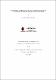# Iterative approximation of solutions of some optimization problems in Banach spaces.2018

## Abstract

Let C be a nonempty closed convex subset of a q-uniformly smooth Banach space X which admits a weakly sequentially continuous generalized duality mapping. In this dissertation, we study the approximation of the zero of a strongly accretive operator A : X ! X which is also a xed point of a k-strictly pseudo-contractive self mapping T of C: Also, we introduce a U-mapping for nite family of mixed equilibrium problems involving 􀀀 relaxed monotone operators. We prove a strong convergence theorem for nding a common solution of nite family of these equilibrium problems in a uniformly smooth and strictly convex Banach space. We present some applications of this theorem and a numerical example. Furthermore, due to the faster rate of convergence of inertial type algorithm, we propose an inertial type iterative algorithm and prove a weak convergence theorem of the scheme to a solution of split variational inclusion problems involving accretive operators in Banach spaces. We give some applications and a numerical example to show the relevance of our result. Our results in this dissertation extend and improve some recent results in the literature.

## Description

Master’s Degree. University of KwaZulu-Natal, Durban.